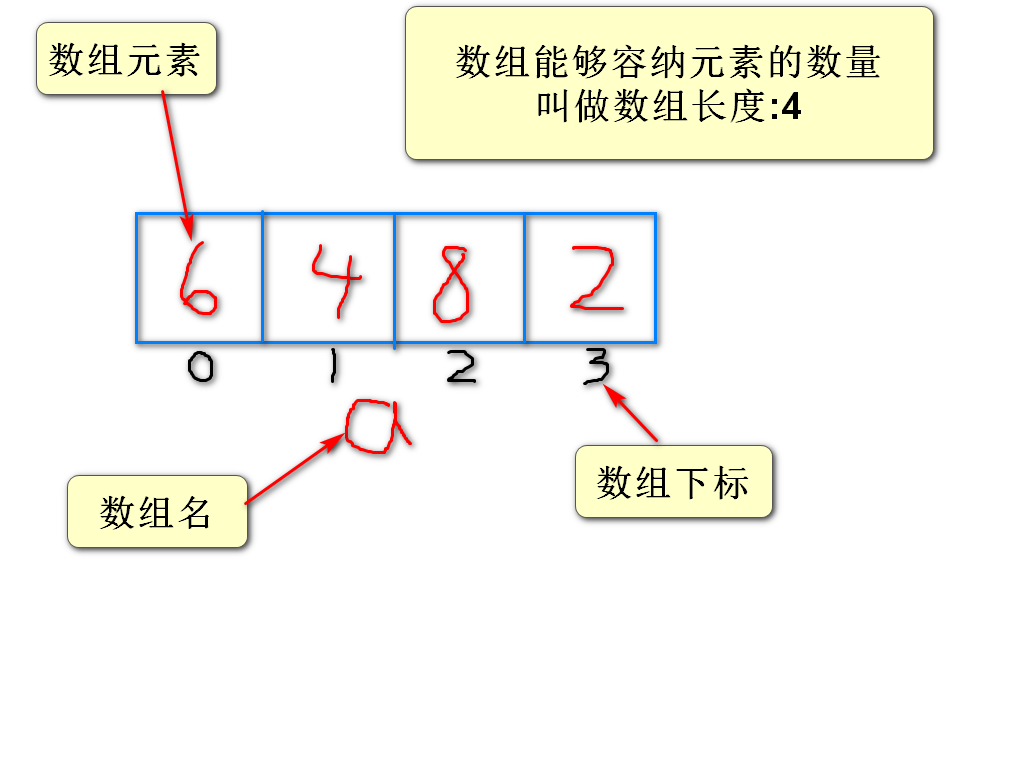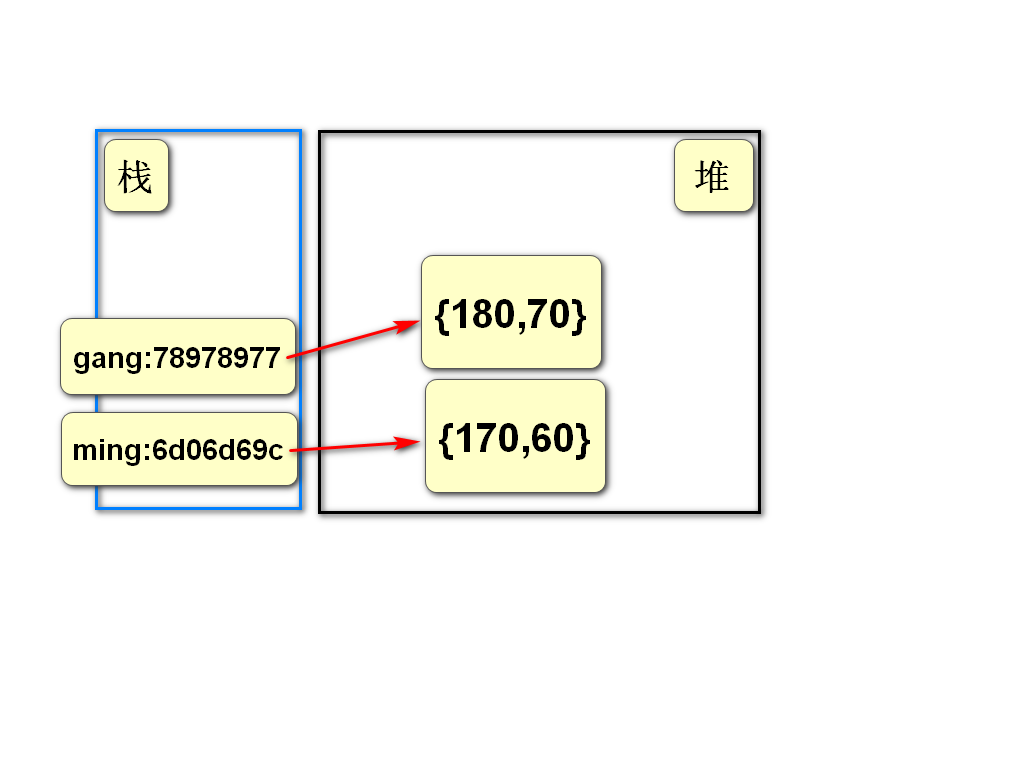## 1.数组

### 3)数组的组成

3.1)数组名称
3.2)数组元素
3.3)数组长度
3.4)数组下标### 4)数组的声明

``````//  <类型>[] <数组名>;
int[] nums;
int nums[];//不推荐``````

### 5)数组的初始化

``````//  <数组名>=new <类型>[<长度>];
nums=new int;
//  合二为一
int[] nums=new int;``````

### 6)数组的使用

``````  // <数组名>[<下标>]=...;
nums=12;
System.out.println(nums);
nums=nums+8;``````

### 8)数组长度的获取

``````<数组名>.length;

``````

## 2.使用数组的注意事项

### 1)数组初始化之后

``````元素都有默认值
(0\0.0\false)``````

## 3.数组声明时赋值;

``````    //1
int[] nums={9,3,6,4,7,1,8};
//2
int[] nums;
nums=new int[]{5,7,1,9,3,6,3,8};  ``````

## 4.数组的引用类型特征

``````当一个数组通过=赋值给另一个数组时## 5.数组复制的方法

Arrays.copyOf()的特性导致 在程序中需要数组扩容或缩容时优先使用该方法

## 6.数组排序

### 2)使用java提供的排序方法

``````Arrays.sort(1);
1.要排序的数组

# 小练习

1) 有5个学生成绩分别为
75,92,63,70,88

2) 有5个商品价格分别为
25.7 , 12.4 , 8.0 , 3.6 , 6.8

3) 定义一个长度为8的数组

## 数组复制

1) int[] num={7,6,3,9,8,4,1,2};

arr : {0,0,9,8,4,0,0};

2) Arrays.copyOf():
int[] nums={1,2,3,4,5,6};

arr: {1,2,3,4,5,6,0,0,0}

### Comment